# Exercise E9.2 Areas of Parallelograms and Triangles NCERT Solutions Class 9

## Chapter 9 Ex.9.2 Question 1

In the given figure, $$ABCD$$ is parallelogram, $$AE ⊥ DC$$ and $$CF ⊥ AD$$ . If $$AB = 16\; \rm{cm}$$, $$AE = 8 \;\rm{cm}$$ and $$CF = 10\;\rm{cm}$$, find $$AD$$.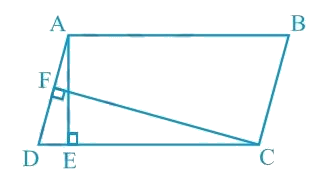### Solution

What is known?

ABCD is parallelogram $${AE}\bot {DC}$$ , and  $${CF}\bot {AD}$$ . If $$AB = 16 \,\rm{cm}, AE = 8 \,\rm{cm}$$ and $$CF = 10\, \rm{cm}$$, find $$AD$$.

What is unknown?

Length of $$AD$$.

Reasoning:

Area of a parallelogram $$ABCD$$

\begin{align}&= CD \!\!\times\!\!{ AE}\,\\&=\,\,{AD}\,\!\!\times\!\!\,{CF}\end{align}

Steps:

Given:

$$AB = 16\; \rm{cm}$$.

$$AE = 8 \;\rm{cm}$$.

$$CF = 10\;\rm{cm}$$.

In parallelogram $$ABCD$$, $$CD = AB = 16 \;\rm{cm}$$

[Opposite sides of a parallelogram are equal]

We know that,

Area of a parallelogram $$=$$ Base $$×$$ Corresponding altitude

Therefore, Area of a parallelogram \begin{align} {\rm{ABCD = CD \times AE}}\,{\rm{ = }}\,\,{\rm{AD}}\,\,{\rm{ \times }}\,\,{\rm{CF}}\end{align}

Here $$CD$$ and $$AD$$ act as base and $$AE ⊥ CD$$ , $$CF ⊥ AD$$ are the corresponding altitudes.

Therefore,\begin{align} {AD} &= \frac{{{{CD }} \times {{ AE}}}}{{{{CF}}}}\end{align}

Substituting the values for $$CD$$ , $$AE$$ and $$CF$$, we get

\begin{align}{AD}&=\frac{16 \times 8}{10} {cm}=12.8 {cm}\end{align}

Thus, the length of $$AD$$ is $$12.8 \;\rm{cm}$$.

## Chapter 9 Ex.9.2 Question 2

If $$E$$, $$F$$, $$G$$ and $$H$$ are respectively the mid-points of the sides of a parallelogram $$ABCD$$ show that $$ar (EFGH)$$ \begin{align}= \frac{1}{2} \end{align}$$\;ar \;(ABCD)$$

### Solution

What is known?

$$E$$, $$F$$, $$G$$ and $$H$$ are respectively the mid-points of the sides of a parallelogram $$ABCD.$$

What is unknown?

How we can show that \begin {align}ar (EFGH) =\frac{1}{2} ar (ABCD).\end{align}

Reasoning:

If a triangle and parallelogram are on same base and between same parallel lines, then area of triangle will be half of area of parallelogram. We can observe in figure that triangle $$GHF$$ and parallelogram $$DHFC$$ are on same base and between parallel lines, Similarly triangle $$EHF$$ and Parallelogram $$AHFB$$ are on same base and between parallel lines. By using these results we can find area and add them to get the required result.

Steps: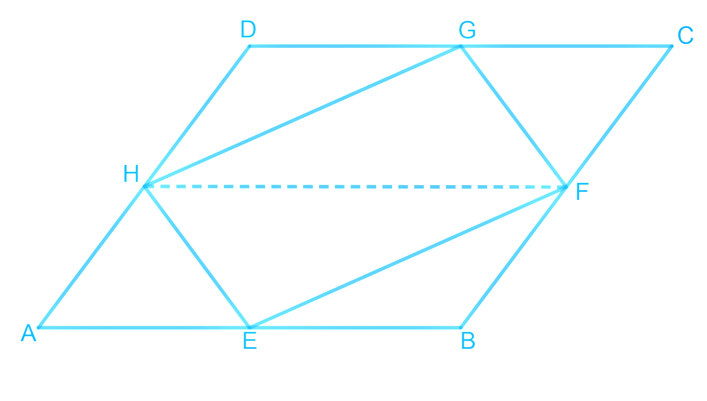Let us join $$HF$$.

In parallelogram $$ABCD$$,

$$AD = BC$$ and $$AD \;|| \;BC$$ (Opposite sides of a parallelogram are equal and parallel).

$$AB = CD$$ (Opposite sides of a parallelogram are equal).

\begin{align}\frac{1}{2} {AD}=\frac{1}{2} {BC} \text { and } {AH} \| {BF}\end{align}

$$AH = BF$$ and $$AH || BF$$ ($$H$$ and $$F$$ are the mid-points of $$AD$$ and $$BC$$)

Therefore, $$ABFH$$ is a parallelogram.

Since $$\Delta\, HEF$$ and parallelogram $$ABFH$$ are on the same base $$HF$$ and between the same parallel lines $$AB$$ and $$HF$$,

\begin{align} \text { Area }(\Delta {HEF})\!=\!{1}{2} \text { Area }({ABFH}) \!\ldots(1)\end{align}

Similarly, it can be proved that

\begin{align}\text { Area}(\Delta {HGF})\!\!=\!\!\frac{1}{2} \text { Area} (HDCF) \!\dots(2)\end{align}

On adding Equations ($$1$$) and ($$2$$), we obtain

\begin{align}&{\rm{Area}}\left( {\Delta{{HEF}}} \right)\!+\!{\rm{Area}}\left( {\Delta {{HGF}}} \right)\\ &\!\!=\!\!\frac{1}{2} {\rm{Area}}\left( {{{ABFH}}} \right)\!+\!\frac{1}{2}{\rm{Area}}\left( {{{HDCF}}} \right)\end{align}

From the figure, we can see that:

\begin{align}&{\text{Area }}\left( {\Delta \,{{HEF}}} \right)+ {\text{ Area }}\left( {\Delta \,{{HGF}}} \right)\\& = {\text{ Area} (EFGH) \text{and} \quad \cdots \cdots (3) }\end{align}

\begin{align}&\frac{1}{2}\,\,{\text{Area }}\left( {{{ABFH}}} \right)+ \frac{1}{2}{\rm{ Area }}\left( {{{HDCF}}} \right)\\&=\frac{1}{2}\,{\text{Area}}\left( {{{ABCD}}} \right) \qquad \cdots \cdots(4)\end{align}

From $$\rm{}(3)$$ And $$\rm{}(4)$$ we have, Area \begin{align}\left( {EFGH} \right)\text{ }=\text{ }\frac{1}{2}\,\text{Area}\left( {ABCD} \right)\end{align}

## Chapter 9 Ex.9.2 Question 3

$$P$$ and $$Q$$ are any two points lying on the sides $$DC$$ and $$AD$$ respectively of a parallelogram $$ABCD$$. Show that $$ar (APB) = ar (BQC)$$.

### Solution

What is known?

$$P$$ and $$Q$$ are any two points lying on the sides $$DC$$ and $$AD$$ respectively of a parallelogram $$ABCD$$.

What is unknown?

How we can show that $$ar (APB) = ar (BQC).$$

Reasoning:

If a triangle and parallelogram are on same base and between same parallel lines, then area of triangle will be half of area of parallelogram. So, we can find parallelogram area by two ways and equate them to get the required result.

Steps: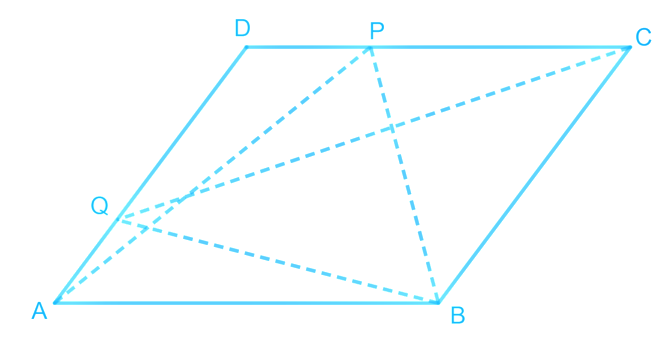It can be observed that $$\Delta BQC$$ and parallelogram $$ABCD$$ lie on the same base $$BC$$ and these are between the same set of parallel lines $$AD$$ and $$BC$$.

\begin{align}\therefore \rm{Area} \left( \Delta \,{BQC} \right)\!\!=\!\!\frac{1}{2}\text{Area }\!\left( {ABCD} \right)\!\!\ldots \left( 1 \right)\end{align}

Similarly, $$\Delta APB$$ and parallelogram $$ABCD$$ lie on the same base $$AB$$ and between the same set of parallel lines $$AB$$ and $$DC$$.

\begin{align}\therefore\!\text { Area }\!(\Delta {APB})\!\!=\!\!\frac{1}{2} \text { Area }\!({ABCD}) \!\cdots(2)\end{align}

From Equations ($$1$$) and ($$2$$), we obtain \begin{align}\rm {Area}\; (\Delta BQC) = Area\; (\Delta APB)\end{align}

## Chapter 9 Ex.9.2 Question 4

In the given figure, $$P$$ is a point in the interior of a parallelogram $$ABCD$$. Show that

(i)

\begin{align}ar (APB)\!+\!ar (PCD)\!=\!\frac{1}{2} ar(ABCD)\end{align}

(ii)

$$ar(APD)\!+\!ar(PBC)\!=\!ar(APB)\!+\!ar(PCD)$$

[Hint: Through. $$P$$, draw a line parallel to $$AB$$]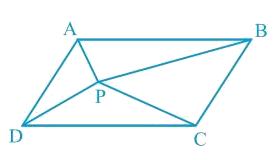### Solution

What is known?

$$P$$ is a point in the interior of a parallelogram $$ABCD.$$

What is unknown?

How we can show that

(i)$$ar(APB)\!+\!ar(PCD)\!=\!\frac{1}{2}ar(ABCD)$$

(ii) $$ar (APD)\!+\!ar(PBC)\!=ar(APB)\!+\!ar(PCD)$$

Reasoning:

Draw a line parallel to and $$CD$$ passes through point $$P$$. Now we can observe the figure, if a triangle and parallelogram are on same base and between same parallel lines then area of triangle will be half of area of parallelogram so we can find area of triangles $$APB$$ and $$PCD$$ to get the required result. Similarly, we can draw a line parallel to $$AD$$ and $$BC$$ to get the required result.

Steps: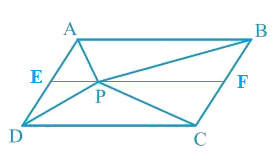(i) Let us draw a line segment $$EF$$, passing through the point $$P$$ and parallel to line segment $$AB$$ in parallelogram $$ABCD$$,

$$AB\; || \;EF$$ (By construction) ... (1)

We know that, $$ABCD$$ is a parallelogram.

$$\therefore AD\; ||\; BC$$ (Opposite sides of a parallelogram are parallel)

$$AE\; || \;BF$$ ... (2)

From Equations (1) and (2), we obtain

$$AB\; || \;EF$$ and $$AE\; || \;BF$$

Therefore, quadrilateral $$ABFE$$ is a parallelogram. Similarly , it can be deduced that quadrilateral $$EFCD$$ is a parallelogram.

It can be observed that $$\Delta APB$$ and parallelogram $$ABFE$$ are lying on the same base $$AB$$ and between the same set of parallel lines $$AB$$ and $$EF$$.

\begin{align}\therefore \!\text{Area}(\Delta {APB})\!=\!\frac{1}{2} \text{Area}({ABFE})\dots(3)\end{align}

Similarly, for $$\Delta PCD$$ and parallelogram $$EFCD$$,

\begin{align}\therefore \rm{Area}(\Delta{PCD})\!=\!\frac{1}{2}\text{Area} (EFCD) \dots(4)\end{align}

Adding Equations ($$3$$) and ($$4$$), we obtain

\begin{align}\begin{bmatrix}\text{Area }(\Delta {APB})+\\ \text { Area }(\Delta{PCD})\end{bmatrix}=\frac{1}{2} \begin{bmatrix}\text { Area }({ABFE})+\\\text { Area }({EFCD})]\end{bmatrix}\end{align}

\begin{align}\begin{bmatrix}\text{Area }\left(\Delta {APB}\right)+\\\text { Area }(\Delta {PCD})\end{bmatrix}=\frac{1}{2}\text { Area }({ABCD})\rm{ ...(5)}\end{align}

(ii)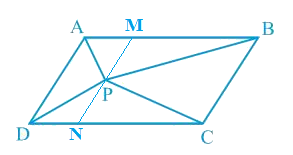Let us draw a line segment $$MN$$, passing through point $$P$$ and parallel to line segment $$AD$$. In parallelogram $$ABCD$$,

$$MN \;|| \;AD$$ (By construction) ... (6)

We know that, $$ABCD$$ is a parallelogram.

$$\therefore$$  $$AB\; ||\; DC$$ (Opposite sides of a parallelogram are parallel)

$$AM\; ||\; DN$$ … (7)

From Equations (6) and (7), we obtain

$$MN \;|| \;AD$$ and $$AM\; ||\; DN$$

Therefore, quadrilateral $$AMND$$ is a parallelogram.

It can be observed that $$\Delta APD$$ and parallelogram  are lying on the same base  and between the same parallel lines  and .

\begin{align}\therefore \text{Area }\!(\Delta {APD})\!=\!\frac{1}{2} \text {Area} (AMND) \ldots(8)\end{align}

Similarly, for $$\Delta PCB$$ and parallelogram ,

\begin{align}\therefore \rm{Area}\,(\Delta {PCB})\!=\!\frac{1}{2}\text { Area }({MNCB})...(9)\end{align}

Adding Equations () and (), we obtain

\begin{align}\begin{bmatrix}\text{Area }\left(\Delta {APD}\right)+\\\text { Area }(\Delta {PCB})\end{bmatrix}= \frac{1}{2}\begin{bmatrix} \text { Area }({AMND})+\\\text { Area }({MNCB})\end{bmatrix}\end{align}

\begin{align}\begin{bmatrix}\text{Area }\left(\Delta {APD}\right)+\\\text { Area }(\Delta {PCB})\end{bmatrix}=\frac{1}{2} \text { Area }({ABCD})...(10) \end{align}

On comparing Equations () and (), we obtain

\begin{align}\begin{bmatrix}\rm{Area}\,\,(\Delta {APD})+\\\text { Area }(\Delta {PBC})\end{bmatrix}=\begin{bmatrix}\text { Area }(\Delta {APB})+\\\text { Area }(\Delta {PCD})\end{bmatrix}\end{align}

## Chapter 9 Ex.9.2 Question 5

In the given figure, $$PQRS$$ and $$ABRS$$ are parallelograms and $$X$$ is any point on side $$BR$$. Show that

(i) \begin{align} \text{ar }\left( \text{PQRS} \right)\text{ = ar }\left( \text{ABRS} \right)\end{align}

(ii)\begin{align} ar \left( \Delta\!\!\text{ }AXS \right)= \frac{\text{1}}{2}ar \left( PQRS \right)\end{align}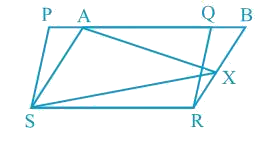### Solution

What is known?

$$PQRS$$ and $$ABRS$$ are parallelograms and X is any point on side $$BR$$.

What is unknown?

How we can show that $$\text{ar }\left( \text{PQRS} \right)\text{ = ar}\left( \text{ABRS} \right)$$ and $$\text{ar }\left( \text{ }\!\!\Delta\!\!\text{ }A\text{XS} \right)\text{ = }\frac{\text{1}}{2}\text{ ar}\left( \text{PQRS} \right)$$

Reasoning:

If a triangle and parallelogram are on same base and between same parallel lines, then area of triangle will be half of area of parallelogram or if two parallelograms are on same and between two parallels lines then their area will be equal. By using these theorems, we can show the required result.

Steps:

(i) It can be observed from the figure that parallelogram $$PQRS$$ and $$ABRS$$ lie on the same base $$SR$$ and also, they lie between the same parallel lines $$SR$$ and $$PB$$.

According to Theorem 9.1: ‘Parallelograms on the same base and between the same parallels are equal in area.'

Therefore

\begin{align} \text{Area }\!( {PQRS})\!=\!\!\text{ Area }\!( {ABRS})\dots({1})\end{align}

(ii) Consider $$\Delta AXS$$ and parallelogram $$ABRS$$.

As Both lie on the same base $$AS$$ and between the same parallel lines $$AS$$ and $$BR$$,

Therefore

\begin{align}\text { Area }\!(\Delta{AXS})\!=\!\frac{1}{2}\,\!\rm{Area} {(ABRS)}\dots(2)\end{align}

From Equations (1) and (2), we obtain

\begin{align} \text{Area }\left( \Delta\!{AXS} \right) = \frac{{1}}{{2}}\text{ Area }\left( {PQRS} \right)\end{align}

## Chapter 9 Ex.9.2 Question 6

A farmer was having a field in the form of a parallelogram $$PQRS$$. She took any point $$A$$ on $$RS$$ and joined it to points $$P$$ and $$Q$$. In how many parts the field is divided? What are the shapes of these parts? The farmer wants to sow wheat and pulses in equal portions of the field separately. How should she do it?

### Solution

What is known?

A farmer was having a field in the form of a parallelogram $$PQRS$$. She took any point A on $$RS$$ and joined it to points P and Q.

What is unknown?

In how many parts the field is divided? What are the shapes of these parts? The farmer wants to sow wheat and pulses in equal portions of the field separately. How should she do it?

Reasoning:

By drawing the figure according to question, we can observe no of parts and shapes of these parts. Also, if a triangle and parallelogram are on same base and between same parallel lines then area of triangle will be half of area of parallelogram.

Steps: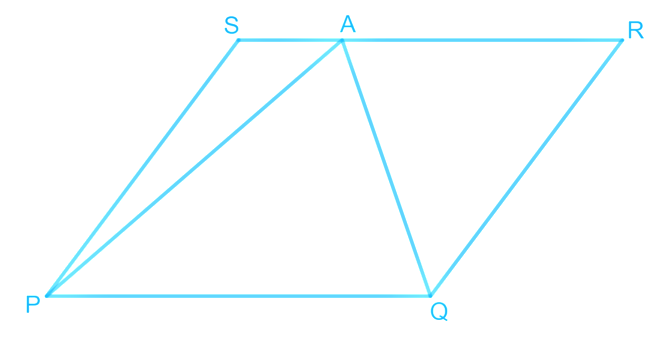From the figure, it can be observed that point $$A$$ divides the field into three parts. These parts are triangular in shape, namely $$\Delta PSA$$, $$\Delta PAQ$$, and $$\Delta QRA$$

From the figure, we can observe that:

\begin{align}\!\begin{bmatrix}\!{\text{Area of}} \Delta PSA+ \\ {\text{ Area of }}\Delta PAQ + \\ \text{Area of }\Delta QRA \!\end{bmatrix}\!=\!\begin{bmatrix} \text{Area of}\\ \text{parallelogram} \\{ PQRS}\end{bmatrix}\ldots (1)\end{align}

We know that if a parallelogram and a triangle are on the same base and between the same set of parallel lines, then the area of the triangle is half the area of the parallelogram.

Therefore

\begin{align}\!\!\text { Area }\!\!(\Delta PAQ)\!=\!\frac{1}{2} \rm{Area} {(PQRS)}....(2)\end{align}

From Equations ($$1$$) and ($$2$$), we obtain

\left[ \begin{align} & \text{Area}\left( \Delta {PSA} \right)+\text{Area}\left( \Delta {QRA} \right) \\& =\frac{1}{2}\text{Area}\left( {PQRS} \right) \\\end{align} \right]...\left( \text{3} \right)

Clearly, it can be observed that the farmer must sow wheat in triangular part $$PAQ$$ and pulses in other two triangular parts $$PSA$$ and $$QRA$$ or wheat in triangular parts $$PSA$$ and $$QRA$$ and pulses in triangular parts $$PAQ$$.

In this way, the farmer can sow wheat and pulses in equal portions of the field separately.

Instant doubt clearing with Cuemath Advanced Math Program